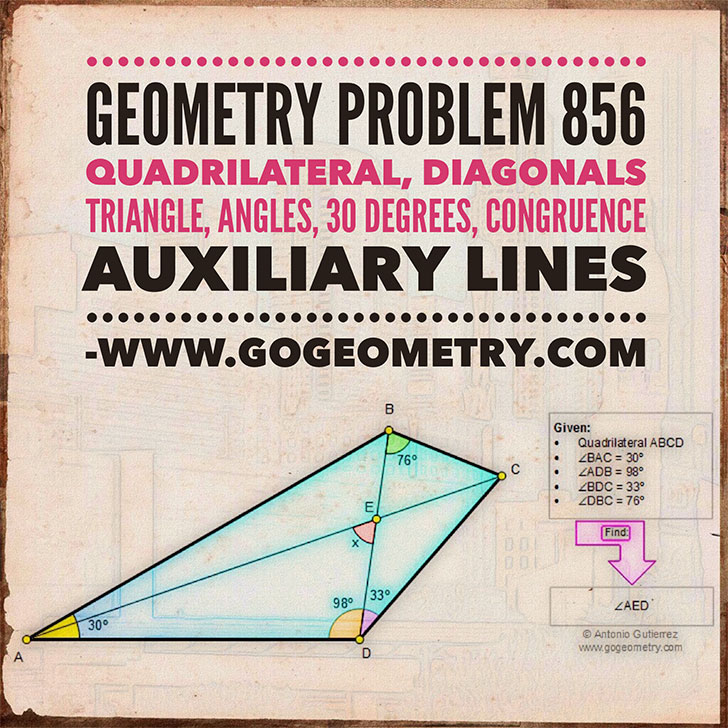# Online Geometry Problem 856: Quadrilateral, Diagonals, Triangle, Angles, 30 degrees, Congruence, Auxiliary lines. Level: High School, SAT Prep, College, Mathematics Education

 The figure below shows a quadrilateral ABCD with angle BAD = 30 degrees, angle ADB = 98 degrees, angle BDC = 33 degrees, and angle DBC = 76 degrees. If the diagonals intersect at E, find the measure of angle AED. Try to use elementary geometry (no trigonometry.# Geometry Problem 856: Typography, Mobile Apps, iPadHome | Search | Geometry | Problems | All Problems | Open Problems | Visual Index | 10 Problems | 851-860 | Triangles | Quadrilaterals | Angles | 30 degrees | Email | Solution / comment | by Antonio Gutierrez